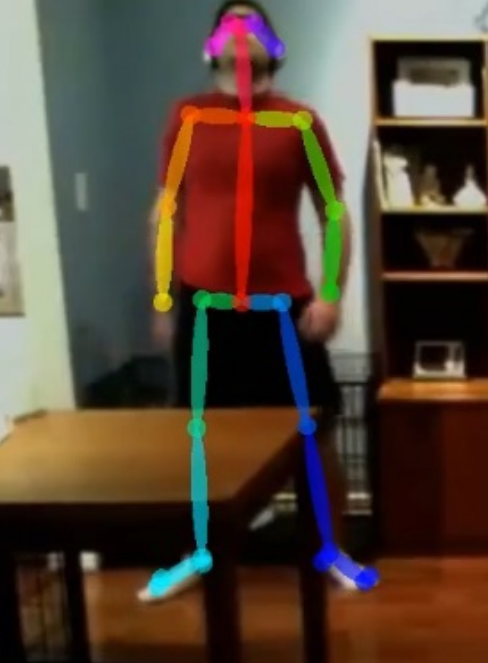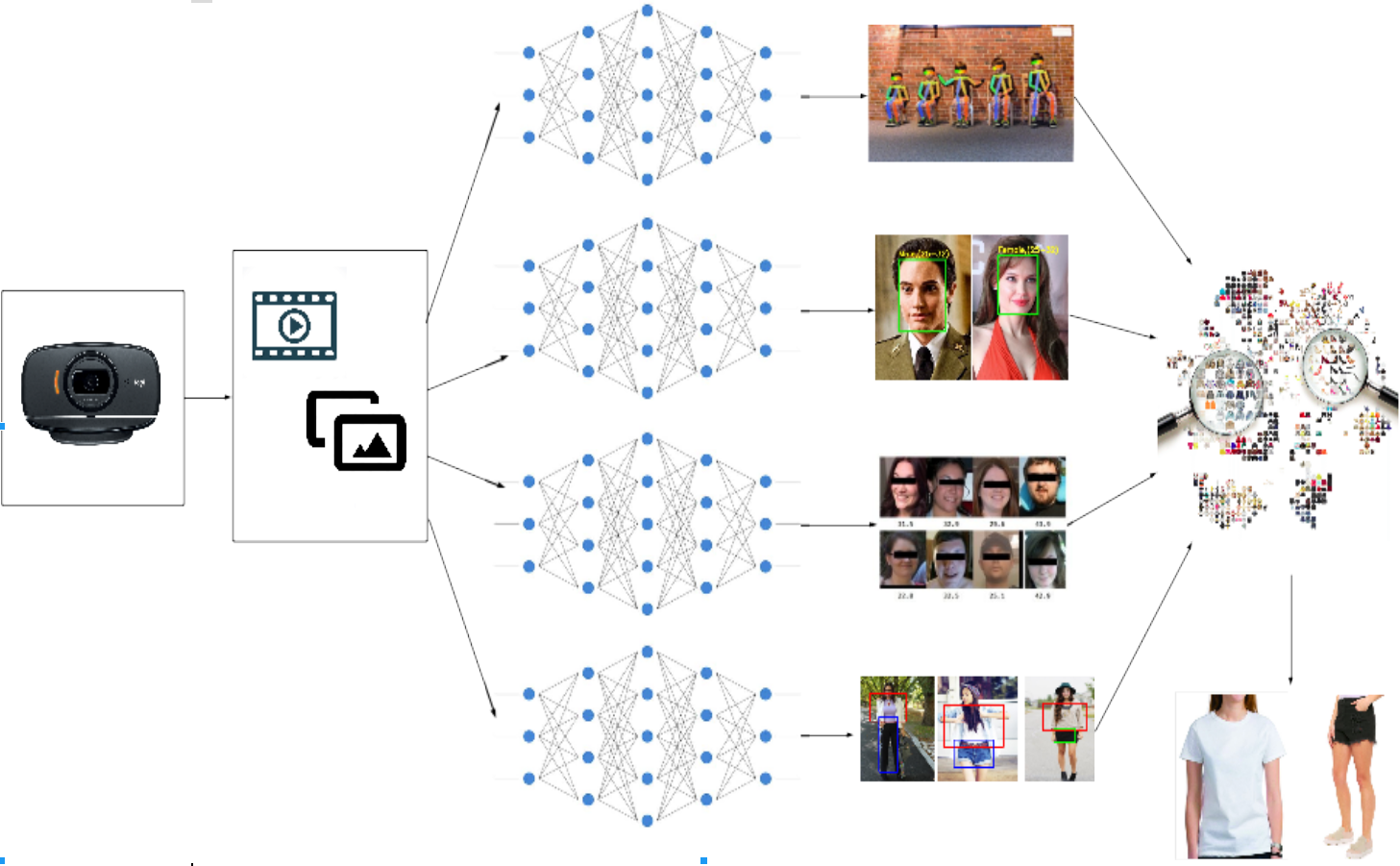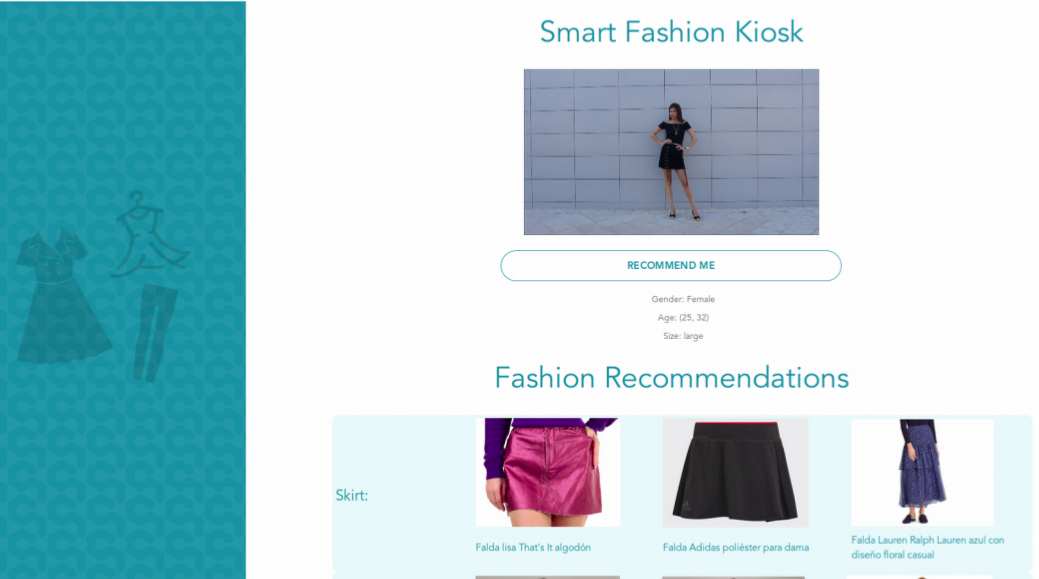{{announcement.body}}
{{announcement.title}}

# Building a Recommendation System Using Deep Learning Models

DZone 's Guide to

# Building a Recommendation System Using Deep Learning Models

### This tutorial explains how we can integrate some deep learning models in order to make an outfit recommendation system.

· AI Zone ·
Free Resource

Comment (0)

Save
{{ articles.views | formatCount}} Views

In this article, I am going to explain how we integrate some deep learning models, in order to make an outfit recommendation system. We want to build an outfit recommendation system. We used four deep learning models to get some important characteristics of the clothing used by the user.

The recommendation systems can be classified into 4 groups:

• Recommendation based on product characteristics
• Recommendation based on the behavior that other users have with the product
• Recommendation based on the user's general characteristics
• Recommendation based on more than one of the previously mentioned criteria

In our case, we are going to make recommendations based on the user and product characteristics. The user characteristics that we took into account were gender, age, and body mass index (BMI). The product characteristics we took into account were the type of clothes the user is wearing. So we need a photograph of the user in order to make the predictions of all the characteristics we need to make the outfit recommendation.

We are going to obtain the garment characteristics from a full-body image of the user.

We use a pose estimator named AlphaPose to determine if the user is complete or not. Alpha Pose detects 19 key points of a person. If it detects at least 17 points, we assume that the person is complete.Image 1: AlphaPose estimation

We retrained one of the most known classifiers on the web, YOLO v3. YOLO is also one of the most accurate image classifiers. The data set, used for the training is a small set of the huge MMLAB data set, DeepFashion.

The other model used was the Dlib, get_frontal_face_detector(). This model is built over 5 HOG filters. The model detects the front view faces and side view faces. This model was chosen because it has great accuracy and is very quick. For age and gender detection, we followed the data science post where they use openCV and a convolutional neural network for the age and gender classifications.

For the IMC estimate, we based in the article “Estimating Body Mass Index from face images using Keras and transfer learning”Image 2: Architecture of the recommendation system

## Models integration

All the code was written in Python3.5 using some computer vision libraries like OpenCV and some deep learning frameworks like Keras.

``````detector = dlib.get_frontal_face_detector()
# Carga de modelos
# CNN
# Boddy Mass Index
model_bmi = get_trained_model()
### Face Detection
img_h, img_w, _ = np.shape(image)
detected = detector(image, 1)
faces
=
np.empty((1,
config.RESNET50_DEFAULT_IMG_WIDTH, 3))
config.RESNET50_DEFAULT_IMG_WIDTH,detection = {}
if len(detected) > 0:
for i, d in enumerate(detected):
x1, y1, x2, y2, w, h = d.left(), d.top(), d.right() + 1, d.bottom() + 1, d.width(),
d.height()
xw1 = max(int(x1 - margin * w), 0)
yw1 = max(int(y1 - margin * h), 0)
xw2 = min(int(x2 + margin * w), img_w - 1)
yw2 = min(int(y2 + margin * h), img_h - 1)
cv2.rectangle(image, (xw1, yw1), (xw2, yw2), (255, 0, 0), 2)
#Get Face
face_img = frame[yw1:yw2, xw1:xw2].copy()
# Estimación
age, gender = get_age_and_gender(face_img, age_net, gender_net)
# Boddy Mass Index
faces[0,:,:,:]=cv2.resize(face_img,(config.RESNET50_DEFAULT_IMG_WIDTH, 3 )) /255.00
bmi = round(float(model_bmi.predict(faces)),2)
detection[i]={'gender':gender, 'age':age, 'bmi':bmi}``````

In these few lines, we load the model to RAM and make the pose estimation. We also cut the area of the image where the face is in order to estimate age, gender, and BMI. Then we make the clothes classification with YOLO and get the type of clothes we’ll recommend.

``````def eval_cloth(img_test, categoria_test, size_test):
filename = './ClothEmbedding/X_reduced2.sav'
X_reduced, hasher, pca, df = joblib.load(filename)
img_c = cv2.resize(img, (80, 80), interpolation=cv2.INTER_CUBIC)
img_data_test = img_c.reshape(-1).astype(np.float32)
img_transformed = hasher.transform(img_data_test.reshape(1, -1))
img_reduced = pca.transform(img_transformed)
# Distancia entre la muestra y la base de datos
dist = [np.linalg.norm(img_reduced-e) for e in X_reduced]
df['distance'] = dist
df_test = df.sort_values(['distance'])
# Se conserva sólo la categiría requerida
df_test = df_test[df_test['categoria2'] == categoria_test]
# Se conservan sólo las tallas requeridas
cat_ns = ['tacones', 'chanclas', 'botas', 'bolsa', 'ropa_interior']
if not(categoria_test in cat_ns):
if (len(size_test) == 2):
true_table = [(size_test in sizes_r or size_test in sizes_r) for sizes_r in df_test['tallas']]
else:
true_table = [size_test in sizes_r for sizes_r in df_test['tallas']]
df_test = df_test[true_table]
return df_test``````

This last function receives all the information of the person and the clothes. The clothing characteristics are compared to the clothes in our database and the recommendations are made by an embedding. We recommend similar clothes to the ones the user is wearing.

And last, thinking in the UX, we made a front end.Image 3: Web view built for the recommendation system

Topics:
ai ,computer vision ,python ,recommendation engine ,deep learning models ,artificial intelligence ,clothing recommendation system

Comment (0)

Save
{{ articles.views | formatCount}} Views

Opinions expressed by DZone contributors are their own.